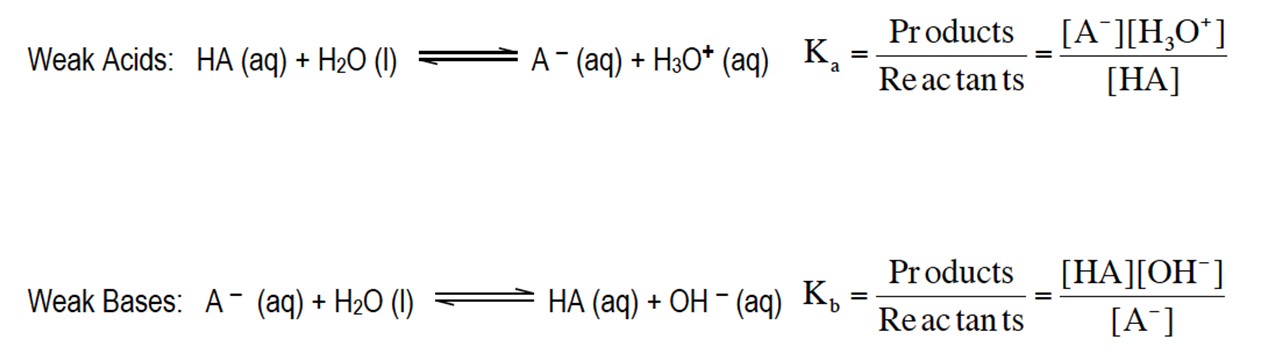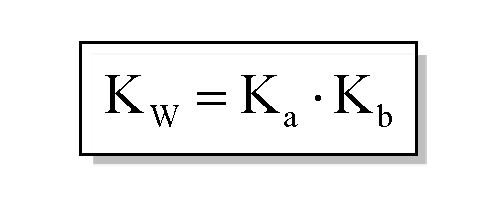Clutch Prep is now a part of Pearson
Ch.10 Acids and BasesWorksheetSee all chapters

# Ka and Kb

See all sections
Sections
Acid-Base Introduction
Arrhenius Acid and Base
Bronsted Lowry Acid and Base
Acid and Base Strength
Ka and Kb
The pH Scale
Auto-Ionization
pH of Strong Acids & Bases
Acid-Base Equivalents
Acid-Base Reactions
Gas Evolution Equations (Simplified)
Ionic Salts (Simplified)
Buffers
Henderson-Hasselbalch Equation
Strong Acid Strong Base Titrations (Simplified)

Associated with any weak acid or weak base is a Ka or Kb value respectively.

###### Acid and Base Dissociation Constants.

Concept #1: Understanding Ka and Kb Expressions.

Transcript

Hey guys, in this new video, we're going to take a look at the acid and base constants. As you guys can know from looking at the rules for identifying compounds as either acids or bases, you should realize that there's a lot more weak species out there than there are strong ones. What we need to realize is these weak species have constants associated with them. We're going to say here consider a weak monoprotic HA acid. Monoprotic just means it has one H positive. You were looking at how it ionizes in water. Basically, HA is the acid so it’s going to give away an H positive to the water. The water accepts the H positive so that’s how it becomes H3O+. The acid loses an H positive and that’s how it becomes A minus. What we're going to say here, the equilibrium expression equals products over reactants. Remember, we’ve talked about the equilibrium expression before. It looks at all phases of matter except for liquids and solids. Liquids and solids will not be included. Here we have a liquid, so we’re going to ignore it when we do the equilibrium expression. It's simply going to become A minus times H3O+ over HA.

Weak acids possess a Ka value less than 1, while weak bases possess a Kb value less than 1. The equilibrium expressions of Ka and Kb are the same as other equilibrium constants we’ve seen.Concept #2: Understanding Ka and Kb.

Transcript

Now, we're going to say that ka represents our acid dissociation constant and it basically measures how strong our weak acids are, and we're going to say weak acids use ka, weak bases don't use ka, instead they use Kb, so this is our base dissociation constant and we're going to say here is their relationship to each other ka and Kb can be expressed by this formula Kw equals ka times Kb and as you realize this Kw is the same Kw we saw earlier in the earlier times, we saw it as Kw equals h positive times OH minus, it's the same exact Kw and we're going to say in general, the higher the ka value then the stronger the acid, the stronger the acid the greater the concentration of H+. Now, we're going to say related to ka is pKa and remember, we've talked about what p means, p means negative log. So, pKa means negative log of ka, here we're going to say there's an inverse relationship, it's actually the lower the pKa the stronger the acid and the stronger the acid the greater the H+ concentration. Now, we're going to say here is when it comes to a weak acid, we're going to say weak acid tends to have Ka value less than 1 and a pKa value greater than 1 just realize, we said inverse relationship. So, k and pKa are opposites, if one is up the other one is down, strong acids though, strong acids tend to have pKa is much greater than 1, I mean not pK's, but Kas much greater than 1 and they tend to have pk's lower than 1, pKa's that are negative and remember, we should be able to establish an a relationship between ka and pKa in the same way we've been able to establish a relationship between H+ and pH because, here we can just simply say ka equals 10 to the negative pKa, we could also say the same thing for pkb, pkb equals negative log of kb and therefore Kb equals 10 to the negative pKa, just remember these relationships and how they affect the acidity of our compound, as long as you can remember these few quick easy calculations you'll be able to go from one to the other and one last thing, we can say here that the relationship from p to pKa and pKb can be established by looking at this equation here, by taking the negative log of Kw ka and Kb, we say that the equation becomes 14 equals pKa plus pKb. Now, that we've seen all these types of new variables and new types of equations attempt to do the practice question on the bottom here, I say if the Kb of NH3 is 1.76 times 10 to the negative 5, what is the acid dissociation constant of the conjugate acid, conjugate acid is a term we learned earlier a few videos back but here we were given Kb, we are asked to find the acid dissociation constant. So, it's a simple conversion from one unit to another. So, once you're done with that click back on the explanation button and see how I approach this problem good luck guys.

The greater the Ka value then the stronger the acid, while the greater the Kb the stronger the base. Ka and Kb are connected by the following equation:Example #1: Knowing that HF has a higher Ka value than CH3COOH, determine, if possible, in which direction the following equilibrium lies.

HF (aq) + CH3COO  (aq) F  (aq) + CH3COOH (aq)

Practice: If the Kb of NH3 is 1.76 x 10-5, determine the acid dissociation constant of its conjugate acid.

Practice: Which Bronsted-Lowry base has the greatest concentration of hydroxide ions?

Practice: Which Bronsted-Lowry acid has the weakest conjugate base?